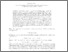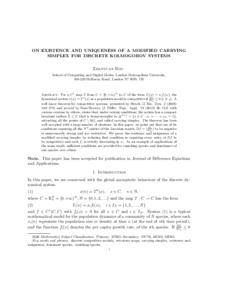# On existence and uniqueness of a modified carrying simplex for discrete Kolmogorov systems

Hou, Zhanyuan (2021) On existence and uniqueness of a modified carrying simplex for discrete Kolmogorov systems. Journal of Difference Equations with Applications, 27 (2). pp. 284-315. ISSN 1023-6198Preview
Text
Carrying_simplex_discrete.pdf - Accepted Version

Official URL: https://doi.org/10.1080/10236198.2021.1894141

## Abstract / Description

For a $C^1$ map $T$ from $C =[0, +\infty)^N$ to $C$ of the form $T_i(x) = x_if_i(x)$, the dynamical system $x(n) =T^n(x)$ as a population model is competitive if $\frac{\partial f_i}{\partial x_j}\leq 0$ $(i\not= j)$. A well know theorem for competitive systems, presented by Hirsch (J. Bio. Dyn. 2 (2008) 169--179) and proved by Ruiz-Herrera (J. Differ. Equ. Appl. 19 (2013) 96--113) with various versions by others, states that, under certain conditions, the system has a compact invariant surface $\Sigma\subset C$ that is homeomorphic to $\Delta^{N-1} =\{x\in C: x_1+ \cdots + x_N=1\}$, attracting all the points of $C\setminus\{0\}$, and called carrying simplex. The theorem has been well accepted with a large number of citations. In this paper, we point out that one of its conditions requiring all the $N^2$ entries of the Jacobian matrix $Df = (\frac{\partial f_i}{\partial x_j})$ to be negative is unnecessarily strong and too restrictive. We prove the existence and uniqueness of a modified carrying simplex by reducing that condition to requiring every entry of $Df$ to be nonpositive and each $f_i$ is strictly decreasing in $x_i$. As an example of applications of the main result, sufficient conditions are provided for vanishing species and dominance of one species over others.

Item Type: Article discrete competitive models; retrotone maps; carrying simplex; existence and uniqueness; dominant species; vanishing species 500 Natural Sciences and Mathematics > 510 Mathematics500 Natural Sciences and Mathematics > 570 Life sciences; biology School of Computing and Digital Media Zhanyuan Hou 19 Feb 2021 09:14 04 Mar 2022 01:58 https://repository.londonmet.ac.uk/id/eprint/6354View Item